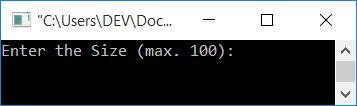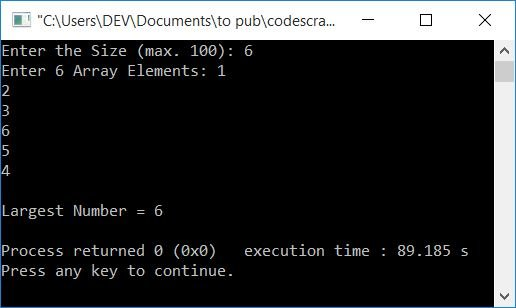# C++ Program to Find the Largest Number in an Array

In this article, you will learn and get code to find and print the largest element or number in an array provided by the user (at run-time) in C++. Here is the list of programs:

## In C++, find the largest element in an array without using a function

To find the largest element in an array in C++ programming, you have to ask the user to enter the size of the array and then enter elements of that size. Then find and print the largest number or element from the given list, as shown in the program given below.

The question is, "Write a program in C++ to find and print the largest number in an array." Here is its answer:

```#include<iostream>
using namespace std;
int main()
{
int arr, tot, larg, i;
cout<<"Enter the Size (max. 100): ";
cin>>tot;
cout<<"Enter "<<tot<<" Array Elements: ";
for(i=0; i<tot; i++)
cin>>arr[i];
larg = arr;
for(i=1; i<tot; i++)
{
if(larg<arr[i])
larg = arr[i];
}
cout<<"\nLargest Number = "<<larg;
cout<<endl;
return 0;
}```

This program was built and runs under the Code::Blocks IDE. Here is its sample run:Now enter the array's size, say 6.And then enter any six numbers or elements. After supplying all the inputs, press the ENTER key to find and print the largest number from the given list of numbers, as shown in the snapshot given below:The statement:

`larg = arr;`

states that it is assumed that the largest element is present at the very first index (that is, 0th) of the array. Or it is supposed that the largest element is the first element of the array. We compared the value of larg with each and every value present at rest indexes. While comparing, if the value of larg is found to be less, then the new value (which is greater than larg's value) gets initialized to it (the larg variable).

The dry run of the above program with user input 6 as size and 1, 2, 3, 6, 5, 4 as 6 array elements goes like this:

• Because the array size is entered as 6. So tot=6
• And when the user enters six array elements, then it gets stored in arr[], in this way:
• arr=1
• arr=2
• arr=3
• arr=6
• arr=5
• arr=4
• The value at the 0th index, 1 is now initialized to larg. So larg=1
• The execution of the for loop starts. That is, its initialization part gets executed first and only once. So i=1
• With i=1, the condition i<tot or 1<6 evaluates to be true, therefore program flow goes inside the loop.
• Inside the loop, the condition larg<arr[i] or 1<arr or 1<2 evaluates to be true, therefore program flow goes inside the if's body and the value of arr[i] gets initialized to larg. So larg=2
• Now the program flow goes to the update part of the for loop and increments the value of i and evaluates the condition again.
• That is, i=2 and the condition i<tot or 2<6 again evaluates to be true, therefore, program flow again goes inside the loop.
• This process continues until the condition is evaluated as false.
• After exiting the loop, the variable larg holds the largest element from the given array.
• As a result, print the value of larg.

## Using a C++ Function, find the largest element in an array

Let's create the same-purpose program using a user-defined function, findLarge(). This function takes two arguments. The first argument is the array, and the second is its size. The largest element gets returned by this function.

```#include<iostream>
using namespace std;
int findLarge(int [], int);
int main()
{
int arr, tot, larg, i;
cout<<"Enter the Size (max. 100): ";
cin>>tot;
cout<<"Enter "<<tot<<" Array Elements: ";
for(i=0; i<tot; i++)
cin>>arr[i];
larg = findLarge(arr, tot);
cout<<"\nLargest Number = "<<larg;
cout<<endl;
return 0;
}
int findLarge(int a[], int s)
{
int i, m;
m = a;
for(i=1; i<s; i++)
{
if(m<a[i])
m = a[i];
}
return m;
}```

This program produces the same output as the previous program.

#### The same program in different languages

C++ Quiz

« Previous Program Next Program »